#Unity在UI界面上显示3D模型/物体，控制模型旋转

1
1
11. 云栖社区>
2. 博客>
3. 正文

## Unity在UI界面上显示3D模型/物体，控制模型旋转

chinar-yunxi 2018-07-16 01:00:14 浏览3950

Unity3D物体在UI界面的显示

 本文提供全流程，中文翻译。 Chinar 坚持将简单的生活方式，带给世人！（拥有更好的阅读体验 —— 高分辨率用户请根据需求调整网页缩放比例）

 Chinar —— 心分享、心创新！助力快速利用 UGUI 完成 3D 物体在 UI 界面的显示为新手节省宝贵的时间，避免采坑！

Chinar 教程效果：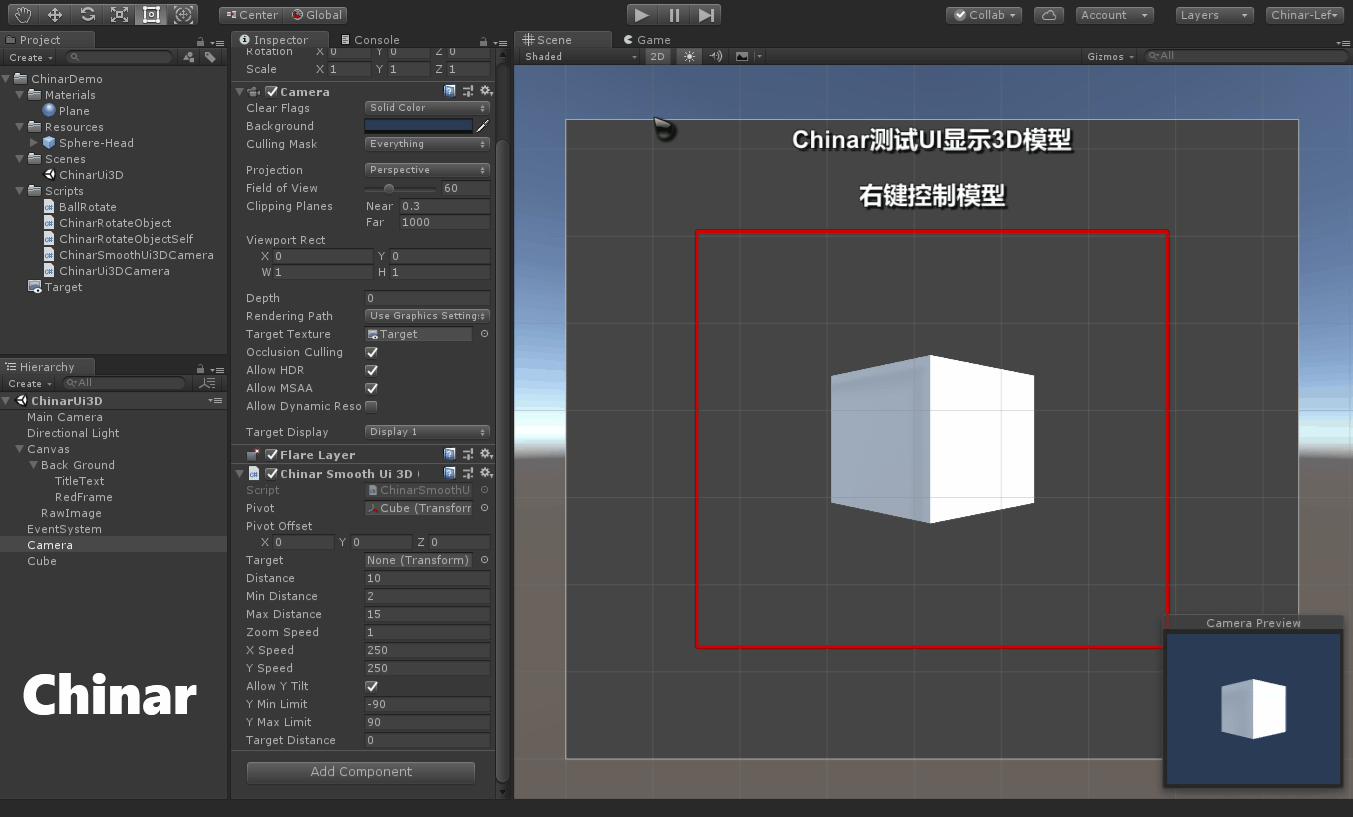# 1

## Description —— 教程描述# 2

## Create UI —— 创建一个UI元素

Hierarchy / 层次面板中，创建一个 UI 元素 RawImage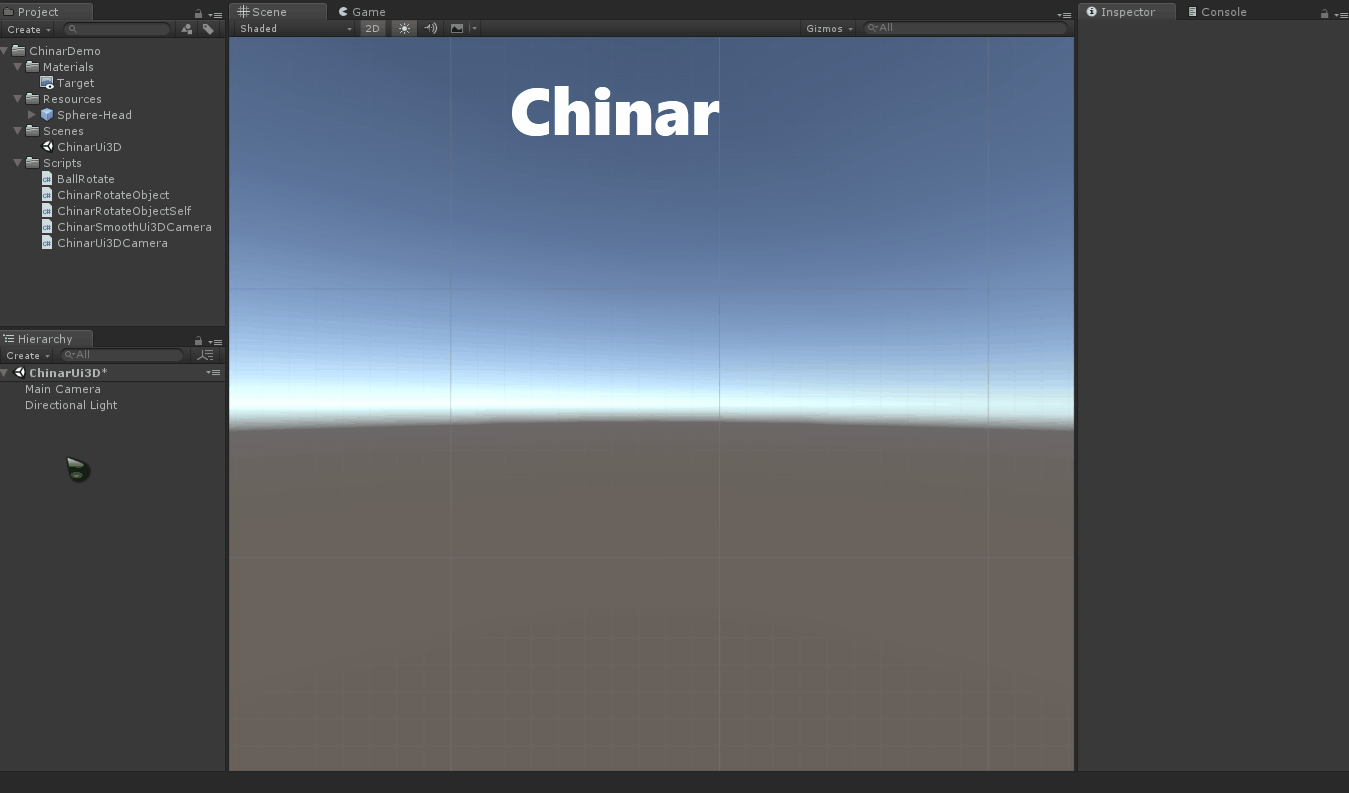# 3

## Create RenderTexture And Camera —— 创建渲染纹理与相机

RenderTexture 是可以设置分辨率的，默认只有256*256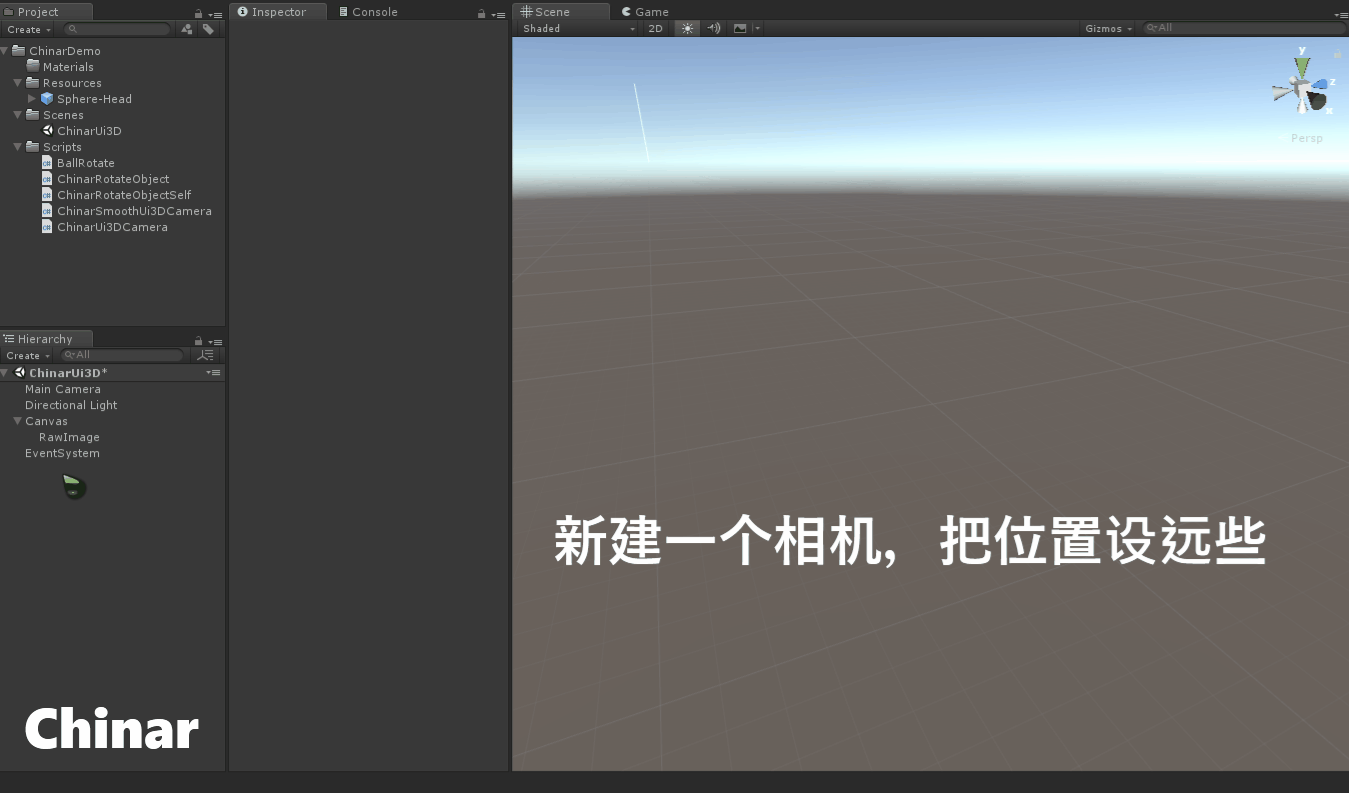# 4

## Relevance RawImage —— 将渲染纹理关联图片

RenderTexture 指定相机后，同样指定给最初创建的 Relevance RawImage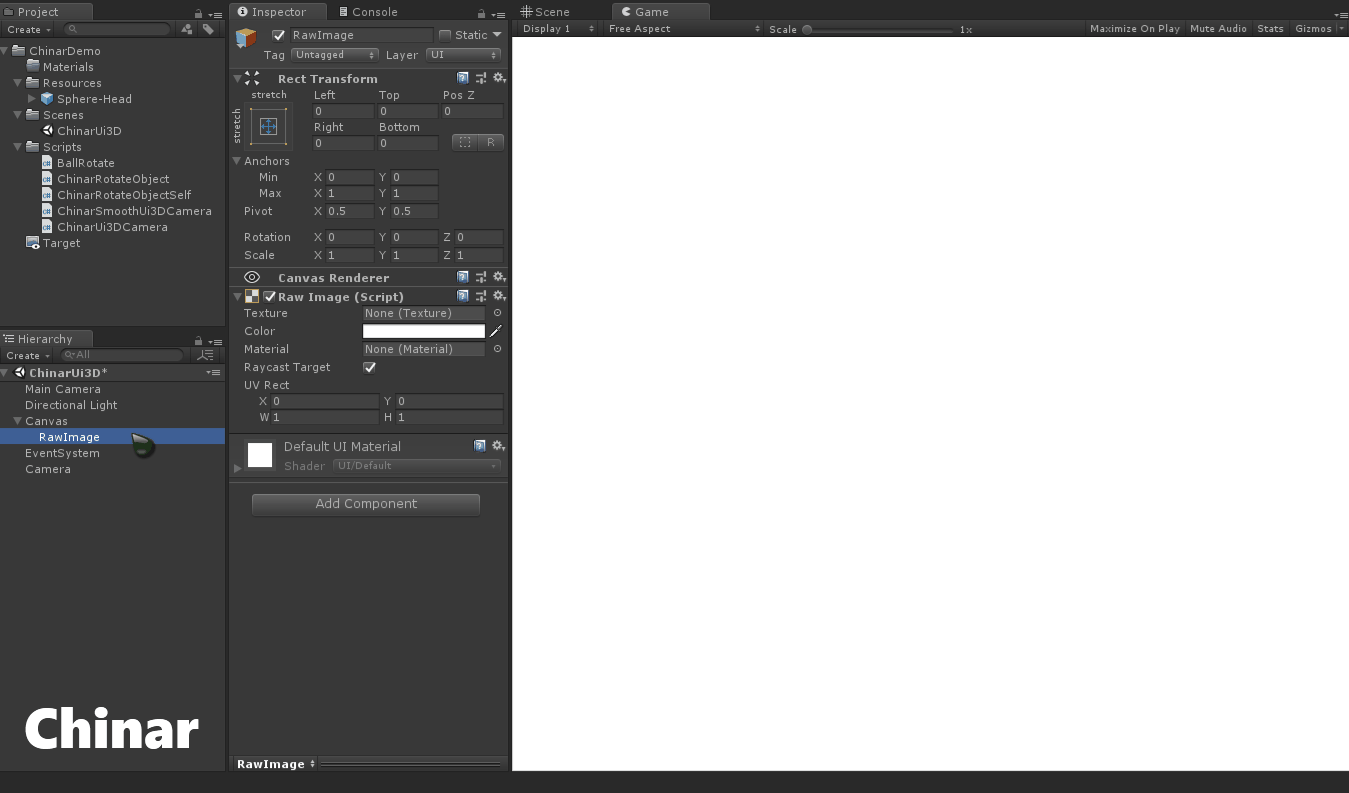# 5

## Create Cube —— 创建一个立方体，看下3D效果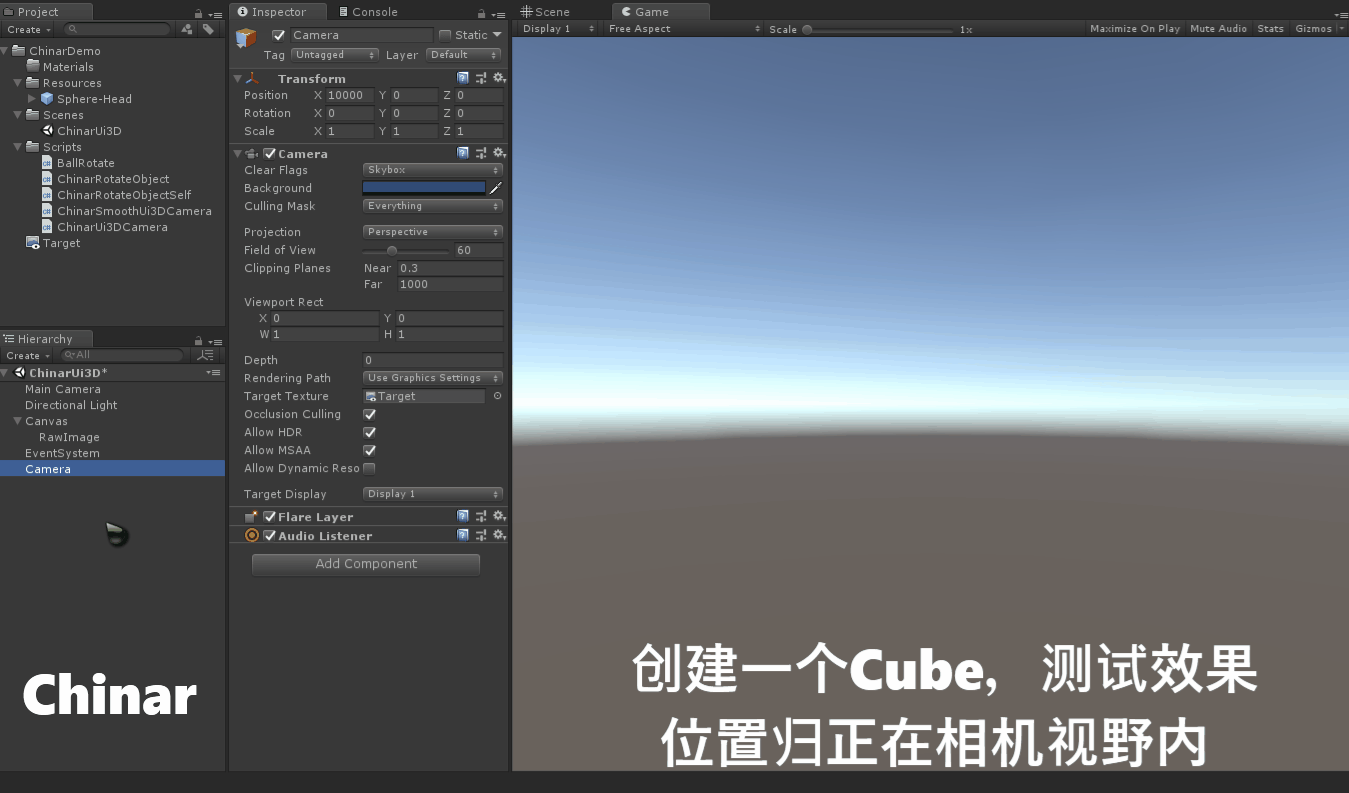# 6

## Control The Rotation —— 控制3D模型/物体旋转

UI 进行整理（这里我只是为了 Demo效果更便于新手理解，大家也可以不做整理依旧出效果）using UnityEngine;

/// <summary>
/// 脚本挂载新建相机上 —— 新相机Clear Flags清除标记设置为：Solid Color，不然会显示天空盒
/// </summary>
public class ChinarSmoothUi3DCamera : MonoBehaviour
{
public  Transform pivot;
public  Vector3   pivotOffset = Vector3.zero;
public  Transform target;
public  float     distance       = 10.0f;
public  float     minDistance    = 2f;
public  float     maxDistance    = 15f;
public  float     zoomSpeed      = 1f;
public  float     xSpeed         = 250.0f;
public  float     ySpeed         = 250.0f;
public  bool      allowYTilt     = true;
public  float     yMinLimit      = -90f;
public  float     yMaxLimit      = 90f;
private float     x              = 0.0f;
private float     y              = 0.0f;
private float     targetX        = 0f;
private float     targetY        = 0f;
public  float     targetDistance = 0f;
private float     xVelocity      = 1f;
private float     yVelocity      = 1f;
private float     zoomVelocity   = 1f;

private void Start()
{
var angles         = transform.eulerAngles;
targetX        = x = angles.x;
targetY        = y = ClampAngle(angles.y, yMinLimit, yMaxLimit);
targetDistance = distance;
}

private void LateUpdate()
{
if (!pivot) return;
var scroll = Input.GetAxis("Mouse ScrollWheel");
if (scroll      > 0.0f) targetDistance -= zoomSpeed;
else if (scroll < 0.0f)
targetDistance += zoomSpeed;
targetDistance = Mathf.Clamp(targetDistance, minDistance, maxDistance);
if (Input.GetMouseButton(1) || (Input.GetMouseButton(0) && (Input.GetKey(KeyCode.LeftControl) || Input.GetKey(KeyCode.RightControl))))
{
targetX += Input.GetAxis("Mouse X") * xSpeed * 0.02f;
if (allowYTilt)
{
targetY -= Input.GetAxis("Mouse Y") * ySpeed * 0.02f;
targetY =  ClampAngle(targetY, yMinLimit, yMaxLimit);
}
}

x = Mathf.SmoothDampAngle(x, targetX, ref xVelocity, 0.3f);
y = allowYTilt ? Mathf.SmoothDampAngle(y, targetY, ref yVelocity, 0.3f) : targetY;
Quaternion rotation = Quaternion.Euler(y, x, 0);
distance = Mathf.SmoothDamp(distance, targetDistance, ref zoomVelocity, 0.5f);
Vector3 position = rotation * new Vector3(0.0f, 0.0f, -distance) + pivot.position + pivotOffset;
transform.rotation = rotation;
transform.position = position;
}

private static float ClampAngle(float angle, float min, float max)
{
if (angle < -360) angle += 360;
if (angle > 360) angle  -= 360;
return Mathf.Clamp(angle, min, max);
}
}

# 7

## Project —— 项目文件

Demo 仅29K# 支持

## May Be —— 搞开发，总有一天要做的事！

 拥有自己的服务器，无需再找攻略！ Chinar 提供一站式教程，闭眼式创建！为新手节省宝贵时间，避免采坑！END

chinar-yunxi
+ 关注